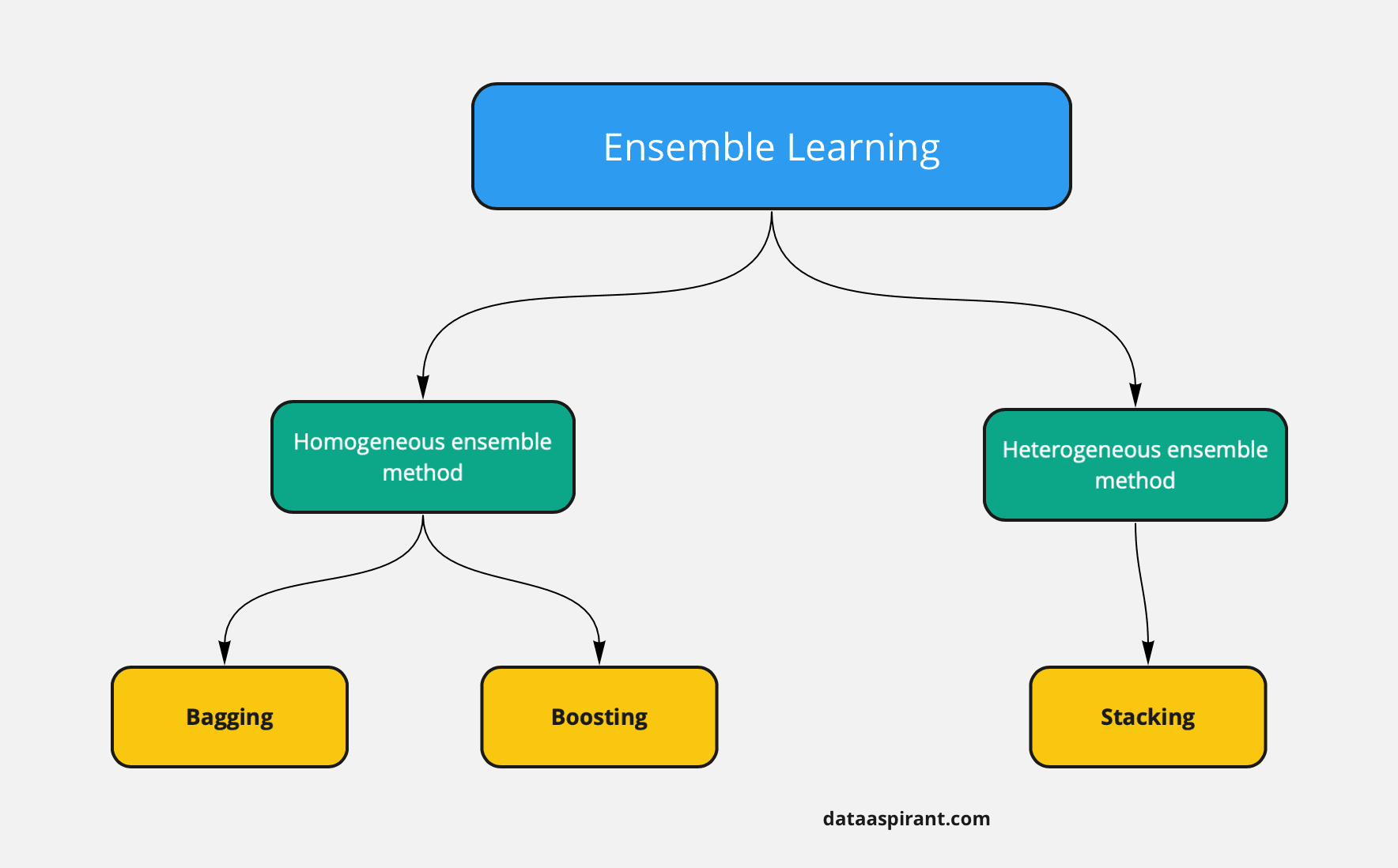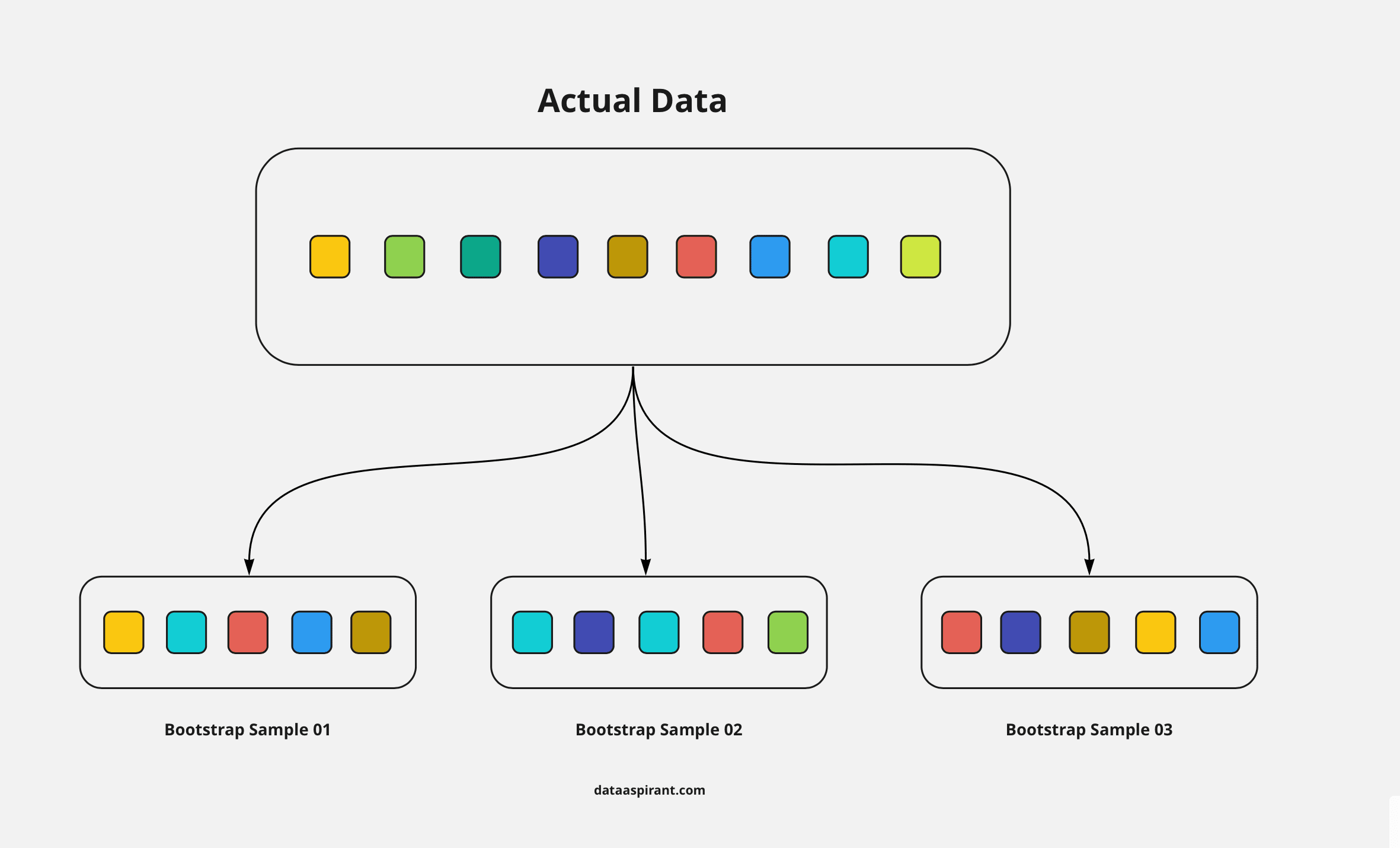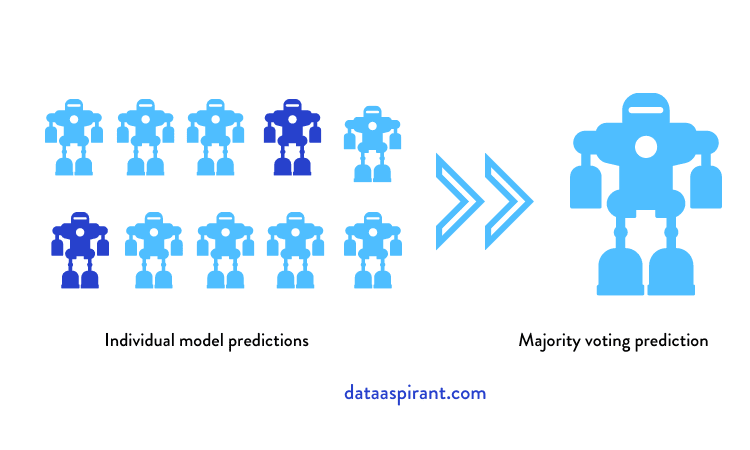## Ensemble Methods | Bagging Vs Boosting Difference

[ad_1]In the world of machine learning, ensemble learning methods are the most popular topics to learn. These ensemble methods have been known as the winner algorithms. In the data science competitions platform like Kaggle, machinehack, HackerEarth ensemble methods are getting hype as the top-ranking people in the leaderboard are frequently using these methods like bagging methods and boosting methods.

For the data scientist roles, in interviews the difference between bagging and boosting most frequently asked question.

So in this article, we are going to learn different kinds of ensemble methods. In particular, we are going to focus more on Bagging and boosting approaches. First, we will walk through the required basic concepts. Later we will learn in-depth about these methods.

Before we drive further, below is the list of concepts you are going to learn in this article.

## What is Ensemble Learning?

In machine learning instead of building only a single model to predict target or future, how about considering multiple models to predict the target. This is the main idea behind ensemble learning.

In ensemble learning we will build multiple machine learning models using the train data, we will discuss how we are going to use the same train data to build various models in the next sections of this article.

So what advantage will we get with ensemble learning?

This is the primary question that will arrive in our mind.

Let’s pass a second here to think about what advantage we will get if we build multiple models.

With a single model approach, if the build model is having high bias or high variance we will be limited to that. Even though we are having methods to handle high bias or high variance. Still if the final is facing any of the bias or variance issues we can’t do anything.

Whereas if we  build multiple models we can reduce the high variance and high bias issue by averaging all models. If the individual models are having high bias, then when we build multiple models the high bias will average out. The same is true for high variance cases too.

For building multiple models we are going to use the same train data.

If we use the same train data, then all the build models will be also the same right?

But this is not the case.

We will learn how to build different models using the same train dataset. Each model will be unique to itself. We will split the available train data into multiple smaller datasets. But while creating these datasets we should follow some key properties. We will talk more about this in the bootstrapping section in this article itself.

For now just remember, to build multiple models we will split the available train data in smaller datasets. In the next steps, we will learn how to build models using the smaller datasets. One model for one smaller dataset.

#### In short:

The ensemble learning means instead of building a single model for prediction. We will build multiple machine learning models, we call these models as weak learners. A combination of all weak learners makes the strong learner, Which  generalizes to predict all the target classes with a decent amount of accuracy.

### Different Ensemble MethodsEnsemble Learning Methods

We are saying we will build multiple models, how these models will differ from one other. We have two possibilities.

• All the models are build using the same machine learning algorithm
• All the models are build using different machine learning algorithms

Based on above mentioned criteria the ensemble methods are of two types.

1. Homogeneous ensemble methods
2. Heterogeneous ensemble methods

Let’s understand these methods individually.

### Homogeneous Ensemble Method

The first possibility of building multiple models is building the same machine learning model multiple times with the same available train data. Don’t worry even if we are using the same training data to build the same machine learning algorithm, still all the models will be different. Will explain this in the next section.

These individual models are called weak learners.

Just keep in mind, in the homogeneous ensemble methods all the individual models are built using the same machine learning algorithm.

For example, if the individual model is a decision tree then one good example for the ensemble method is random forest.

In the random forest model, we will build N different models. All individual models are decision tree models. If you want to learn how the decision tree and random forest algorithm works. Have a look at the below articles.

Both bagging and boosting belong to the homogeneous ensemble method.

### Heterogeneous Ensemble Method

The second possibility for building multiple models is building different machine learning models. Each model will be different but uses the same training data.

Here also the individual models are called weak learners. The stacking method will fall under the heterogeneous ensemble method. In this article, we are mainly focusing only on the homogeneous ensemble methods. In the upcoming articles, we will learn about the staking method.

For now, let’s focus only on homogeneous methods.

By now we are clear with different types of ensemble methods. We frequently said the individual models are weak learners. So let’s spend some time understanding the weak learners and strong learners. These are the building blocks for ensemble methods.

## Weak Learners Vs Strong Learners

In both, homogeneous and heterogeneous ensemble methods we said the individual models are called weak learns, in the homogeneous ensemble method these weak learns are built using the same machine learning algorithms, Whereas in the heterogeneous ensemble methods these weak learns are built using different machine learning algorithms.

So what do these weak learners do? Why are they more important for understanding any ensemble methods?

Weak learning is the same as any machine learning model, unlike the strong machine learning models they won’t try to generalize for all the possible target cases. The weak learners only try to predict a combination of target cases or a single target accurately.

Confusing?

Let’s understand this with an example. Before that, we need to understand about bootstrapping. Once we learn about bootstrapping, then we will take an example to understand weak learning and strong learning methodology in more detail.

### Bootstrapping

For building multiple models whether it is a homogeneous or heterogeneous ensemble method the dataset is the same.

So how to use the same dataset for building multiple models?Bootstrapping Example

For each model, we need to take a sample of data, but we need to be very careful while creating these samples of data. Because if we randomly take the data, in a single sample we will end up with only one target class or the target class distribution won’t be the same. This will affect model performance.

To overcome this we need a smart way to create these samples, known as bootstrapping samples.

Bootstrapping is a statistical method to create sample data without leaving the properties of the actual dataset. The individual samples of data called bootstrap samples.

Each sample is an approximation for the actual data. These individual sample has to capture the underlying complexity of the actual data. All data points in the samples are randomly taken with replacement

In the above image from the actual dataset, we created 3 bootstrap samples. In this case, we are creating an equal size sample. We don’t have any hard rule saying all the bootstrap sample sizes should be the same.

In the bootstrapping properties, we said the data points will take randomly and with replacement. From the above image, the second bootstrapping sample is having a repeated data point (which is light green.)

Same as the above image, using the actual dataset we will create bootstrap samples. Then each bootstrap sample is used to create multiple models.

By now we learned how the individual sample datasets are created and we also learned these datasets are used for building the multiple weak learns. The combination of all weak learns makes a strong learner or strong model.

### Weak LearnersWeak Learners Example

Let’s understand about weak learning with the help of the above example.

Week learns are the individual models to predict the target outcome. But these models are not the optimal models. In other words, we can say they are not generalized to predict accurately for all the target classes and for all the expected cases.

They will focus on predicting accurately only for a few cases. If you see the above example.

The original dataset is having two possible outcomes:

The above representation predicts the target circle or diamonds with some features.

The first learner accurately predicted the circles, the second weak learner also accurately predicting the circles. Whereas the last weak learner is accurately predicting diamonds.  As we said before, weak learning accurately predicts one target class.

Combining all the weak learners makes the strong model which generalized and optimized well enough for accurately predicting all the target classes.

So how do these strong learners work?

### Strong Learning

We said a combination of all the weak learners builds a strong model. How do these individuals build trains at once, how do they perform the predictions?

Based on the way the individual models (weak learners) training phase the bagging and boosting methods will vary.

## What Is Bagging?Bagging Ensemble Method

In the bagging method, all the individual models are built parallel, each individual model is different from one other. In this method, all the observations in the bootstrapping sample will be treated equally. In other words, all the observations will have equal at zero weightage. Because of this bagging method also called bootstrap aggregating.

As a first step using the bootstrapping method, we will split the dataset into N number of samples. Then we will select the algorithm we want to try.

Suppose if we selected a decision tree, then each bootstrap sample will be used for building one random forest model. Don’t forget all the decision trees are built in parallel.

Once the training phase is completed, to predict the target outcome, we will pass the observations to all the N decision trees. Each decision tree will predict one target outcome. The final prediction target will be selected based on the majority voting.Bagging Majority Voting

Suppose we build 10 decision tree models. The target is a binary target. Let’s say the target class could be 1 or 0. Then each decision tree will predict 1 or 0. Out of 10 decision trees, 8 trees are predicted as 1, and 2 trees predicted as 0 by majority voting means the final predicted class will be 1.

Let’s say in the above image out of 10 models 8 models are  predicted one target class and the other 2 models predicted the other target class. So the final predicted target will be the 8 models target, this is known as majority voting.

The bagging methods can be used for both classification and regression problems. If we are using the bagging method of classification method, we use the majority voting approach for the final prediction. Whereas for the regression kind of problems, we take the average of all the values predicted by individual models.

#### Pros

• Bagging helps in reducing the overfitting. As we are averaging all the models outputs using the majority voting approach.

#### Cons

• For regression models the predicted value won’t be optimised. if any one of the models is deviating more as the output value will be average of all the models.

### Bagging Algorithms

Below are the list algorithms that fall under bagging.

## What is Boosting?Boosting Ensemble Method

In the boosting method, all the individual models are built sequentially. Which means the outcome of the first model passes to the next model and etc.

In bagging the models are built parallel so we don’t know what the error of each model is. Whereas in boosting once the first model built we know the error of that model. So when we pass this first model to the next model the intention is to reduce the error further. In some boosting algorithm, each model has to reduce a minimum of 50% of error.

Unlike bagging all the observations in the bootstrapping sample are not equally treated in boosting. Observations will have some weightage. For a few observations, the weightage will be high for others lower.

Suppose we are building a binary classification model. The first model is not accurately predicting the  class 01 target, then the input to the second model will get in sequentially way, saying focus more on predicting target class 01.

When selecting the data samples from the bootstrap sample few observations will have high weightage, in this case the data point which can help in accurately predicting the target class 01 will have higher weightage than the other data points.

For the final target, the predictions from all the models will be weighted. Hence the final prediction will be the weighted average.

#### In Short:

In boosting all the individual models will build one after the other. Each model output will pass as input to the next model along with next model bootstrap sample data.

### Boosting ensemble method Pros & Cons

#### Pros

• Boosting reduces the bias, As each model tries to reduce the errors of the previous model in the sequential chain.

• We can use multiple loss functions to reduce the error in each sequential models.

#### Cons

• Boosting method tends to over-fitting, As the models build sequentially, all the models try to reduce the train error.

• We need special care in turning the hyper-parameters.

### Boosting Algorithms

Below is the list of algorithms that fall under boosting.

• Adaboost algorithm
• Xgbm algorithm
• LightGBM
• CatBoost
• LPBoost
• GradientBoost
• BrownBoost

## Bagging Vs Boosting ComparisonDifference Between Bagging and Boosting

We learned the bagging and boosting separately. Now let’s compare these two ensemble methods to take our understanding to the next level.

### Splitting the datasets

For splitting the actual train data to multiple datasets, as known as the bootstrap samples both these methods use the bootstrapping statistical method.

In bagging once the bootstrap samples create, there will be no changes for building multiple models. where as in the boosting based on the previous model output the individual observation will have weightage. Some data points the bootstrap will have low weightage, whereas some data points will have higher weightage.

### Training individual models (weak learners)

In the training phase both these methods will change in the way they build models. In the bagging method all the individual models will take the bootstrap samples and create the models in parallel. Whereas in the  boosting each model will build sequentially. The output of the first model (the erros information) will be pass along with the bootstrap samples data.

### Performing predictions

For performing in the bagging method, all the individual models will predict the target outcome, using the majority voting approach we will select the final prediction. Whereas in the boosting method all the model predictions will have some weightage, the final prediction will be the weighted average. In the bagging method it is just the normal average.

### Which one is better ? Boosting Or Bagging ?

Now the final question

Which one to choose for modeling then ?

Yes this is the correct way of thinking, where both these ensemble methods are powerful which one we need to choose?

This depends on the problem. Sometimes for selecting the final method we need to have a look at each method’s advantages and disadvantages.

Let’s say if the individual models are getting low model performance then in the bagging the combination of all the low performance models will lead to the low performing model.

Whereas if the individual models are ovefitting then the final model with the boosting method will lead to an overfitting model, in such case we can use the bagging method.

So the final conclusion is we don’t have any hard rule for which method to use but in most cases bagging methods will outperform well than the boosting methods. The main problem with boosting methods is that they tend to overfit the data.

## Conclusion

We learned how bagging and boosting methods are different by understanding ensemble learning. In this process, we learn about bootstrapping, weak learner’s concepts. In the end we learnt how these methods vary in each level of modeling.

[ad_2]

Source link